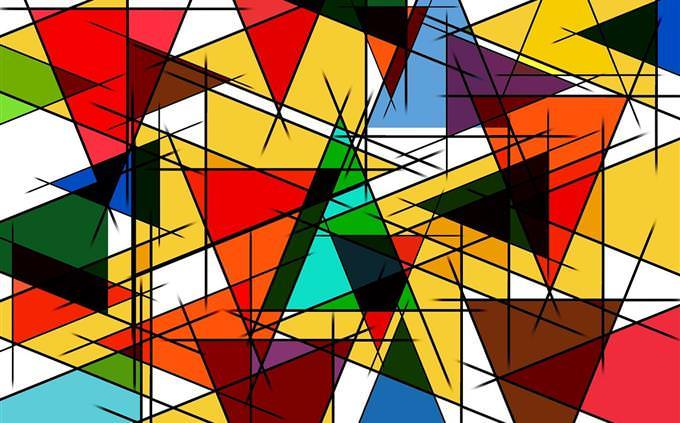Can You Solve These Math Questions?

Subjects like Math and Geometry are considered the most difficult and challenging in the eyes of many, but if you think your mind is strong enough to deal with these fields, you have come to the right place! The following questions will challenge you in a variety of areas related to knowledge as well as computational and logical abilities. Believe that you will be able to answer all 12 successfully? Prove it!We'll start you easy: What is a third of three quarters?
1/4
1/3
1/5
5/12In an equilateral triangle all angles are…
60 degree angles
80 degree angles
90 degree angles
70 degree anglesWhich of these number is not a prime number?
10
2
11
101When 2 regular game dice are rolled, what is the probability that the addition of the 2 numbers obtained will be 12?
1 in 36
1 in 24
1 in 12
1 in 48Solve this equation.
7
21
18
19If 4/7 of a number is 84, what is the whole sum (7/7)?
147
156
184
167What is the sum of the internal angles of a pentagon?
540
360
720
500A particular school has 720 boys and 700 girls. The chance to randomly select a boy learning French is ⅔ and the chance to randomly select a girl learning French is ⅗. How many students learn French in school in total?
900
866
975
850Solve this equation.
12
16
10
6A chocolate cake recipe includes 2 cups of sugar, how many cups of sugar do you need to make 6 and a half cakes?C.M. de Talleyrand-Périgord.

Which of these numbers can be divided by 9 to get a whole number?
333
976
610
372What is the value of 1 to the power of 0?
1
-1
0
IncalculableTry Again
Your result suggests some knowledge gaps worth narrowing down. To do this we recommend that you try again to face this test, or take a look at the correct answers and try to learn a thing or two from them. If you want to try some other challenges, look at some of our other math tests.